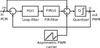# Teledyne DALSAPWM Sigma-Delta Modulator

## Suppliers

Company
Product
DescriptionPWM Sigma-Delta Modulator
A PWM sigma-delta modulator is a special type of 1-bit sigma-delta modulator that produces a pulse width modulated (PWM) output signal with a fixed repetition frequency. A fixed repetition frequency makes the output signal insensitive to typical problems associated with (continuous time) sigma-delta converters, such as non-linear inter symbol interference (ISI) and kickback noise on the reference of the DAC. This silicon-proven IP block implements a digital PWM sigma-delta modulator consisting of a fifth-order loop filter, followed by a 16 taps moving average filter, a sawtooth carrier injection (with a frequency of fCLK/16) and a 1-bit quantizer.

A PWM sigma-delta modulator is a special type of 1-bit sigma-delta modulator that produces a pulse width modulated (PWM) output signal with a fixed repetition frequency. A fixed repetition frequency makes the output signal insensitive to typical problems associated with (continuous time) sigma-delta converters, such as non-linear inter symbol interference (ISI) and kickback noise on the reference of the DAC.
This silicon-proven IP block implements a digital PWM sigma-delta modulator consisting of a fifth-order loop filter, followed by a 16 taps moving average filter, a sawtooth carrier injection (with a frequency of fCLK/16) and a 1-bit quantizer.

## Technical Specifications

Teledyne DALSA
Product Category IP Cores
Product Name PWM Sigma-Delta Modulator
Type Modulator
Unlock Full Specs